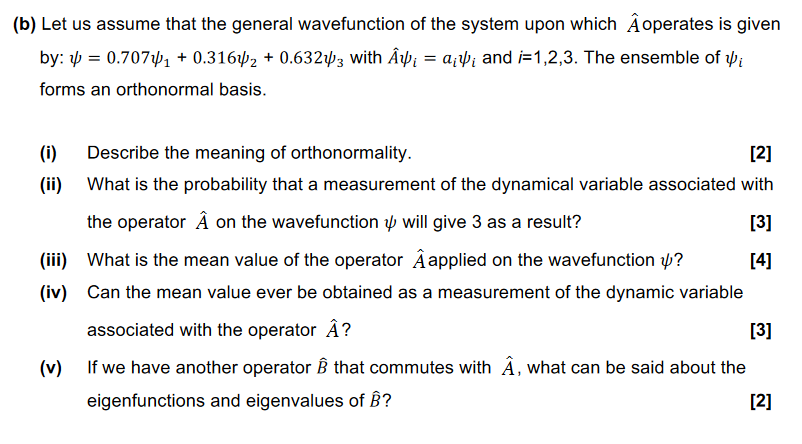Home / Expert Answers / Physics / b-let-us-assume-that-the-general-wavefunction-of-the-system-upon-which-hat-a-operates-is-pa760

# (Solved): (b) Let us assume that the general wavefunction of the system upon which $$\hat{A}$$ operates is ...(b) Let us assume that the general wavefunction of the system upon which $$\hat{A}$$ operates is given by: $$\psi=0.707 \psi_{1}+0.316 \psi_{2}+0.632 \psi_{3}$$ with $$\hat{A} \psi_{i}=a_{i} \psi_{i}$$ and $$i=1,2,3$$. The ensemble of $$\psi_{i}$$ forms an orthonormal basis. (i) Describe the meaning of orthonormality.  (ii) What is the probability that a measurement of the dynamical variable associated with the operator $$\hat{A}$$ on the wavefunction $$\psi$$ will give 3 as a result?  (iii) What is the mean value of the operator $$\hat{A}$$ applied on the wavefunction $$\psi$$ ?  (iv) Can the mean value ever be obtained as a measurement of the dynamic variable associated with the operator $$\hat{A}$$ ?  (v) If we have another operator $$\hat{B}$$ that commutes with $$\hat{A}$$, what can be said about the eigenfunctions and eigenvalues of $$\hat{B}$$ ? 

We have an Answer from Expert